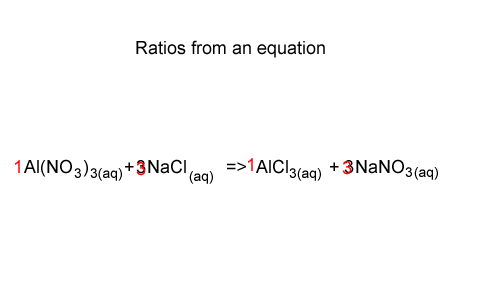Stoichiometry Stoichiometry can be described as the calculation of reactant and product used and produced during a chemical reaction. At the heart of every stoichiometric calculation is the balanced chemical equation. A balanced chemical equation gives us the ratio in which reactants are used and products are produced. Consider the chemical reaction between aluminium nitrate and sodium chloride, shown on the right. The equation shows that aluminium nitrate reacts with sodium chloride in the ratio 1 mole of Al(NO3)3 to 3 mole of NaCl. We can also see that the ratio between NaCl and NaNO3 is 3 : 3 or 1: 1. So for every mole of NaCl used 1 mole of NaNO3 is formed.If we know the number of mole of any product or reactant in a chemical equation then we can work out the number of mole of any other reactant or product. Continue